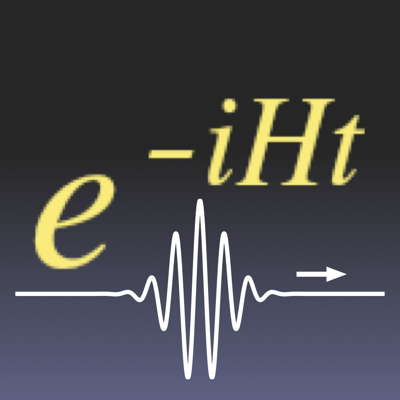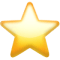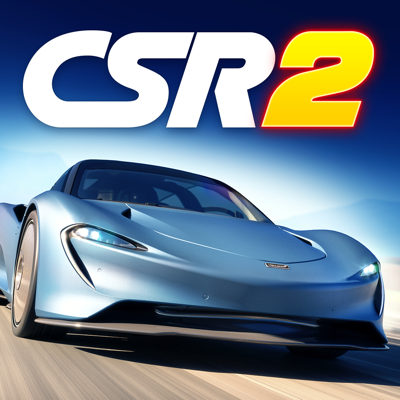Search app# Quantum Wave in a Box

(1)
Price
\$2.99
Category
Education
Last Update
Jan 27, 2017
View in store

#### Store Performance Index

64%

Store Performance Index shows overall performance of your app on app stores. It is based on combined ASO & reviews metrics.

See more data

## Ratings & Reviews performance for Quantum Wave in a Box

Ratings & Reviews performance provides an overview of what users think of your app. Here are the key metrics to help you identify how your app is rated by users and how successful is your review management strategy.

Number of reviews,
total

1

Avg rating,
total2.0

#### Description

3149 chars

Schrödinger equation solver 1D. User defined potential V(x). Diagonalization of hamiltonian matrix. Animation showing evolution in time of a gaussian wave-packet.<br><br>In Quantum Mechanics the one-dimensional Schrödinger equation is a fundamental academic though exciting subject of study for both students and teachers of Physics. A solution of this differential equation represents the motion of a non-relativistic particle in a potential energy field V(x). But very few solutions can be derived with a paper and pencil.<br><br>Have you ever dreamed of an App which would solve this equation (numerically) for each input of V(x) ?<br><br>Give you readily energy levels and wave-functions and let you see as an animation how evolves in time a gaussian wave-packet in this particular interaction field ?<br><br>Quantum Wave in a Box does it ! For a large range of values of the quantum system parameters.<br><br>Actually the originally continuous x-spatial differential problem is discretized over a finite interval (the Box) while time remains a continuous variable. The time-independent Schrödinger equation H ψ(x) = E ψ(x), represented by a set of linear equations, is solved by using quick diagonalization routines. The solution ψ(x,t) of the time-dependent Schrödinger equation is then computed as ψ(x,t) = exp(-iHt) ψ₀(x) where ψ₀(x) is a gaussian wave-packet at initial time t = 0.<br><br>You enter V(x) as RPN expression, set values of parameters and will get a solution in many cases within seconds ! <br><br>- Atomic units used throughout (mass of electron = 1)<br>- Quantum system defined by mass, interval [a, b] representing the Box and (real) potential energy V(x).<br>- Spatially continuous problem discretized over [a, b] and time-independent Schrödinger equation represented by a system of N+1 linear equations using a 3, 5 or 7 point stencil; N being the number of x-steps. Maximum value of N depends on device’s RAM: up to 4000 when computing eigenvalues and eigenvectors, up to 8000 when computing eigenvalues only.<br>- Diagonalization of hamiltonian matrix H gives eigenvalues and eigenfunctions. When computing eigenvalues only, lowest energy levels of bound states (if any) with up to 10-digit precision.<br>- Listing of energy levels and visualisation of eigenwave-functions.<br>- Animation shows gaussian wave-packet ψ(x,t) evolving with real-time evaluation of average velocity, kinetic energy and total energy.<br>- Toggle between clockwise and counter-clockwise evolution of ψ(x,t).<br>- Watch Real ψ, Imag ψ or probability density |ψ|².<br>- Change initial gaussian parameters of the wave-packet (position, group velocity, standard deviation), enter any time value, then tap refresh button to observe changes in curves without new diagonalization. This is particularly useful to get a (usually more precise) solution for any time value t when animation is slower in cases of N being large.<br>- Watch both solution ψ(x,t) and free wave-packet curves evolve together in time and separate when entering non-zero potential energy region.<br>- Zoom in and out any part of the curves and watch how ψ(x,t) evolve locally.

Why to reply to reviews?

User reviews affect conversion to installs and app rating. Featured and helpful reviews are the first to be noticed by users and in case of no response can affect download rate. This is why it is highly recommended to reply to them.

bendyboy76
May 29, 2018

Used to work great. Now crashes when loading and dumps back to home screen in one second.#### About Quantum Wave in a Box

Developed by Michel Ramillon.

Quantum Wave in a Box is ranking in   Education

Last update was at Jan 27, 2017and the current version is 1.0.2.

To see all other keys and revenue click here 1179258292

Quantum Wave in a Box have a 1a user reviews.

Quantum Wave in a Box was released in the App Store. It is developed by Michel Ramillon, who have also released the following apps.

Quantum Wave in a Box has 1 user reviews.​

Quantum Wave in a Box has an average rating of 2.0. ​The latest version of Quantum Wave in a Box 1.0.2​ ​​was ​released on Jan 27, 2017​.

You can download Quantum Wave in a Box ​here